# Complex Numbers Quiz

 Complex Numbers

 Multiple Choice Identify the choice that best completes the statement or answers the question. Simplify.

1.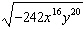a.c.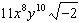b.d.2.a.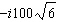c.b.d.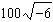3.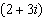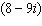a.c.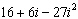b.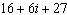d.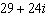4.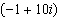a.c.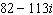b.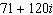d.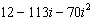5.

What is the sum (14 – 5i) + (8 + 12i)?
 a. 22 – 17i c. 2 – 13i b. 6 + 7i d. 6 – 17i

6.

Which represents the product of 3i and 9 – 5i?
 a. 15 – 27i c. –15 – 27i b. 15 + 27i d. –15 + 27i

7.

Which is equivalent to (7 – 6i)(2 + i)?
 a. 20 – 5i c. 8 + 5i b. 8 – 5i d. 20 + 5i

8.

Which product is a real number?
 a. (3 + 4i)2 c. (3 – 4i)(4 + 3i) b. (3 – 4i)2 d. (3 – 4i)(3 + 4i)

9.

Which is equal to i7?
 a. 1 c. i b. –1 d. –i

10.

Which is the reciprocal of i11?
 a. 1 c. i b. –1 d. –i

11.

Which is equal to (4 – 12i) – (10 – 8i) + (4 + 7i)?
 a. –2 + 3i c. –10 – 13i b. –10 + 3i d. –8 – 13i

12.

Which is equal to?
 a. –41i c. 9 b. –9i d. 41

13.

Which is equivalent to i–30?
 a. 1 c. i b. –1 d. –i

1. D
2. B
3. A
4. C
5. B
6. C
7. A
8. D
9. D
10.C
11.B
12.A
13.B

Source